Courses

# Solid Vapour Equilibrium Civil Engineering (CE) Notes | EduRev

## Civil Engineering (CE) : Solid Vapour Equilibrium Civil Engineering (CE) Notes | EduRev

The document Solid Vapour Equilibrium Civil Engineering (CE) Notes | EduRev is a part of the Civil Engineering (CE) Course Thermodynamics.
All you need of Civil Engineering (CE) at this link: Civil Engineering (CE)

Solid-Vapour Equilibrium

This section deals with the solubility of solids in gases. The phase equilibria consideration here are relatively straightforward as the solubility of gases in solids is usually negligible. Therefore, the solid phase may be considered as pure; all non-ideality in the system therefore, derives from that of the gas phase. In the following analysis we, thus, consider that a pure solid phase (1) co-exists in equilibrium with a vapour-phase mixture of the gas (or solvent, 2) and the solute (i.e., 1). The phase equilibria relation for the component 1 may be written as: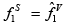....(9.78)

A relation of the form of eqn. 6.119 used for pure liquid phase fugacity calculation may be employed to compute the fugacity of a pure solid at the temperature T and pressure P of the system.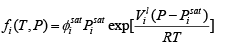....(6.119)

For a pure solid the corresponding equation is: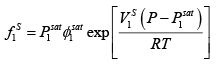....(9.79)

Here V1S is the molar volume of the solid, and P1sat is the vapour-phase saturation pressure for the solid solute at the given temperature. Now for the vapour phase fugacity of the solute on has: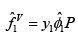....(9.80)

Thus using eqns. 9.78 – 9.80 one obtains: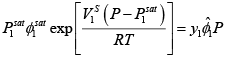....(9.81)
The above equation may be written in a compressed form as follows: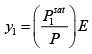....(9.82)

Where,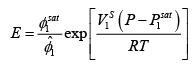....(9.83)

The term E is called the enhancement factor. The vapour pressure P1sat of solids is typically very low (~ 10-5 – 10-3 bar), hence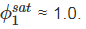Further if the system pressure is also low or as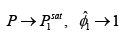Thus, on inspecting eqn. 9.82, it is evident that under such a condition E = 1. The corresponding solubility y1 is termed the ideal solubility. At high pressures (with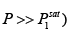, the exponential Poynting factor tends to be greater than one, and the values of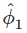are lower than unity. As a result, the factor E typically is higher than one. The enhancement factor, therefore, provides a measure of the degree to which pressure augments the solubility of the solid in the gas phase.

Offer running on EduRev: Apply code STAYHOME200 to get INR 200 off on our premium plan EduRev Infinity!

## Thermodynamics

56 videos|92 docs|33 tests

,

,

,

,

,

,

,

,

,

,

,

,

,

,

,

,

,

,

,

,

,

;